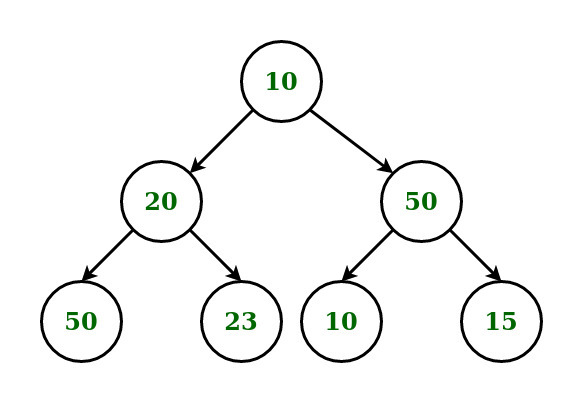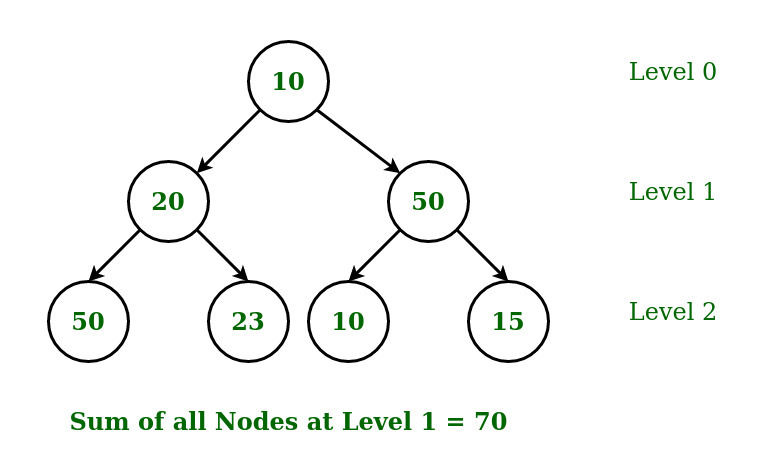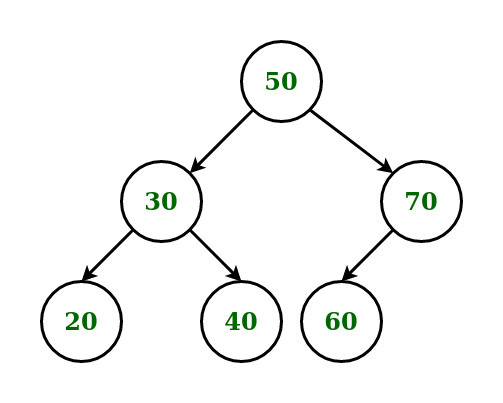# Sum of all nodes at Kth level in a Binary Tree

• Difficulty Level : Easy
• Last Updated : 22 Jun, 2021

Given a binary tree with N nodes and an integer K, the task is to find the sum of all the nodes present at the Kth level.
Examples:

Input:K = 1
Output: 70Input:K = 2
Output: 120

Approach:

• Traverse the Binary Tree using Level Order Traversal and queue
• During traversal, pop each element out of the queue and push it’s child (if available) in the queue.
• Keep the track of the current level of the Binary tree.
• To track the current level, declare a variable level and increase it whenever a child is traversed from the parent.
• When the current level of the tree i.e. the variable level meets the required Kth level, pop the elements from the queue and calculate their sum.

Below is the implementation of the above approach:

## C++

 `// C++ implementation of the approach` `#include ``using` `namespace` `std;` `// Binary tree node consists of data, a``// pointer to the left child and a``// pointer to the right child``struct` `node {``    ``int` `data;``    ``struct` `node* left;``    ``struct` `node* right;``};` `// Function to create new Binary Tree node``struct` `node* newNode(``int` `data)``{``    ``struct` `node* temp = ``new` `struct` `node;``    ``temp->data = data;``    ``temp->left = nullptr;``    ``temp->right = nullptr;``    ``return` `temp;``};` `// Function to return the sum of all``// the nodes at Kth level using``// level order traversal``int` `sumOfNodesAtNthLevel(``struct` `node* root,``                         ``int` `k)``{` `    ``// If the current node is NULL``    ``if` `(root == nullptr)``        ``return` `0;` `    ``// Create Queue``    ``queue<``struct` `node*> que;` `    ``// Enqueue the root node``    ``que.push(root);` `    ``// Level is used to track``    ``// the current level``    ``int` `level = 0;` `    ``// To store the sum of nodes``    ``// at the Kth level``    ``int` `sum = 0;` `    ``// flag is used to break out of``    ``// the loop after the sum of all``    ``// the nodes at Nth level is found``    ``int` `flag = 0;` `    ``// Iterate the queue till its not empty``    ``while` `(!que.empty()) {` `        ``// Calculate the number of nodes``        ``// in the current level``        ``int` `size = que.size();` `        ``// Process each node of the current``        ``// level and enqueue their left``        ``// and right child to the queue``        ``while` `(size--) {``            ``struct` `node* ptr = que.front();``            ``que.pop();` `            ``// If the current level matches the``            ``// required level then calculate the``            ``// sum of all the nodes at that level``            ``if` `(level == k) {` `                ``// Flag initialized to 1``                ``// indicates that sum of the``                ``// required level is calculated``                ``flag = 1;` `                ``// Calculating the sum of the nodes``                ``sum += ptr->data;``            ``}``            ``else` `{` `                ``// Traverse to the left child``                ``if` `(ptr->left)``                    ``que.push(ptr->left);` `                ``// Traverse to the right child``                ``if` `(ptr->right)``                    ``que.push(ptr->right);``            ``}``        ``}` `        ``// Increment the variable level``        ``// by 1 for each level``        ``level++;` `        ``// Break out from the loop after the sum``        ``// of nodes at K level is found``        ``if` `(flag == 1)``            ``break``;``    ``}``    ``return` `sum;``}` `// Driver code``int` `main()``{``    ``struct` `node* root = ``new` `struct` `node;` `    ``// Tree Construction``    ``root = newNode(50);``    ``root->left = newNode(30);``    ``root->right = newNode(70);``    ``root->left->left = newNode(20);``    ``root->left->right = newNode(40);``    ``root->right->left = newNode(60);``    ``int` `level = 2;``    ``int` `result = sumOfNodesAtNthLevel(root, level);` `    ``// Printing the result``    ``cout << result;` `    ``return` `0;``}`

## Java

 `// Java implementation of the approach``import` `java.util.*;` `class` `GFG``{` `// Binary tree node consists of data, a``// pointer to the left child and a``// pointer to the right child``static` `class` `node``{``    ``int` `data;``    ``node left;``    ``node right;``};` `// Function to create new Binary Tree node``static` `node newNode(``int` `data)``{``    ``node temp = ``new` `node();``    ``temp.data = data;``    ``temp.left = ``null``;``    ``temp.right = ``null``;``    ``return` `temp;``};` `// Function to return the sum of all``// the nodes at Kth level using``// level order traversal``static` `int` `sumOfNodesAtNthLevel(node root,``                                ``int` `k)``{` `    ``// If the current node is null``    ``if` `(root == ``null``)``        ``return` `0``;` `    ``// Create Queue``    ``Queue que = ``new` `LinkedList<>();` `    ``// Enqueue the root node``    ``que.add(root);` `    ``// Level is used to track``    ``// the current level``    ``int` `level = ``0``;` `    ``// To store the sum of nodes``    ``// at the Kth level``    ``int` `sum = ``0``;` `    ``// flag is used to break out of``    ``// the loop after the sum of all``    ``// the nodes at Nth level is found``    ``int` `flag = ``0``;` `    ``// Iterate the queue till its not empty``    ``while` `(!que.isEmpty())``    ``{` `        ``// Calculate the number of nodes``        ``// in the current level``        ``int` `size = que.size();` `        ``// Process each node of the current``        ``// level and enqueue their left``        ``// and right child to the queue``        ``while` `(size-- >``0``)``        ``{``            ``node ptr = que.peek();``            ``que.remove();` `            ``// If the current level matches the``            ``// required level then calculate the``            ``// sum of all the nodes at that level``            ``if` `(level == k)``            ``{` `                ``// Flag initialized to 1``                ``// indicates that sum of the``                ``// required level is calculated``                ``flag = ``1``;` `                ``// Calculating the sum of the nodes``                ``sum += ptr.data;``            ``}``            ``else` `{` `                ``// Traverse to the left child``                ``if` `(ptr.left != ``null``)``                    ``que.add(ptr.left);` `                ``// Traverse to the right child``                ``if` `(ptr.right != ``null``)``                    ``que.add(ptr.right);``            ``}``        ``}` `        ``// Increment the variable level``        ``// by 1 for each level``        ``level++;` `        ``// Break out from the loop after the sum``        ``// of nodes at K level is found``        ``if` `(flag == ``1``)``            ``break``;``    ``}``    ``return` `sum;``}` `// Driver code``public` `static` `void` `main(String[] args)``{``    ``node root = ``new` `node();` `    ``// Tree Construction``    ``root = newNode(``50``);``    ``root.left = newNode(``30``);``    ``root.right = newNode(``70``);``    ``root.left.left = newNode(``20``);``    ``root.left.right = newNode(``40``);``    ``root.right.left = newNode(``60``);``    ``int` `level = ``2``;``    ``int` `result = sumOfNodesAtNthLevel(root, level);` `    ``// Printing the result``    ``System.out.print(result);``}``}` `// This code is contributed by 29AjayKumar`

## Python3

 `# Python3 implementation of the approach` `# Binary tree node consists of data, a``# pointer to the left child and a``# pointer to the right child``class` `newNode :``    ``def` `__init__(``self``, data) :``        ``self``.data ``=` `data;``        ``self``.left ``=` `None``;``        ``self``.right ``=` `None``;` `# Function to return the sum of all``# the nodes at Kth level using``# level order traversal``def` `sumOfNodesAtNthLevel(root, k) :` `    ``# If the current node is NULL``    ``if` `(root ``=``=` `None``) :``        ``return` `0``;` `    ``# Create Queue``    ``que ``=` `[];` `    ``# Enqueue the root node``    ``que.append(root);` `    ``# Level is used to track``    ``# the current level``    ``level ``=` `0``;` `    ``# To store the sum of nodes``    ``# at the Kth level``    ``sum` `=` `0``;` `    ``# flag is used to break out of``    ``# the loop after the sum of all``    ``# the nodes at Nth level is found``    ``flag ``=` `0``;` `    ``# Iterate the queue till its not empty``    ``while` `(``len``(que) !``=` `0``) :` `        ``# Calculate the number of nodes``        ``# in the current level``        ``size ``=` `len``(que);` `        ``# Process each node of the current``        ``# level and enqueue their left``        ``# and right child to the queue``        ``while` `(size !``=` `0``) :``            ` `            ``size ``-``=` `1``;``            ``ptr ``=` `que[``0``];``            ``que.pop(``0``);``            ` `            ``# If the current level matches the``            ``# required level then calculate the``            ``# sum of all the nodes at that level``            ``if` `(level ``=``=` `k) :``                ` `                ``# Flag initialized to 1``                ``# indicates that sum of the``                ``# required level is calculated``                ``flag ``=` `1``;``                ` `                ``# Calculating the sum of the nodes``                ``sum` `+``=` `ptr.data;``                ` `            ``else` `:``                ``# Traverse to the left child``                ``if` `(ptr.left) :``                    ``que.append(ptr.left);``                    ` `                ``# Traverse to the right child``                ``if` `(ptr.right) :``                    ``que.append(ptr.right);` `        ``# Increment the variable level``        ``# by 1 for each level``        ``level ``+``=` `1``;` `        ``# Break out from the loop after the sum``        ``# of nodes at K level is found``        ``if` `(flag ``=``=` `1``) :``            ``break``;``    ` `    ``return` `sum``;` `# Driver code``if` `__name__ ``=``=` `"__main__"` `:` `    ``# Tree Construction``    ``root ``=` `newNode(``50``);``    ``root.left ``=` `newNode(``30``);``    ``root.right ``=` `newNode(``70``);``    ``root.left.left ``=` `newNode(``20``);``    ``root.left.right ``=` `newNode(``40``);``    ``root.right.left ``=` `newNode(``60``);``    ``level ``=` `2``;``    ``result ``=` `sumOfNodesAtNthLevel(root, level);` `    ``# Printing the result``    ``print``(result);` `# This code is contributed by AnkitRai01`

## C#

 `// C# implementation of the approach``using` `System;``using` `System.Collections.Generic;` `class` `GFG``{` `// Binary tree node consists of data, a``// pointer to the left child and a``// pointer to the right child``class` `node``{``    ``public` `int` `data;``    ``public` `node left;``    ``public` `node right;``};` `// Function to create new Binary Tree node``static` `node newNode(``int` `data)``{``    ``node temp = ``new` `node();``    ``temp.data = data;``    ``temp.left = ``null``;``    ``temp.right = ``null``;``    ``return` `temp;``}` `// Function to return the sum of all``// the nodes at Kth level using``// level order traversal``static` `int` `sumOfNodesAtNthLevel(node root,``                                ``int` `k)``{` `    ``// If the current node is null``    ``if` `(root == ``null``)``        ``return` `0;` `    ``// Create Queue``    ``List que = ``new` `List();` `    ``// Enqueue the root node``    ``que.Add(root);` `    ``// Level is used to track``    ``// the current level``    ``int` `level = 0;` `    ``// To store the sum of nodes``    ``// at the Kth level``    ``int` `sum = 0;` `    ``// flag is used to break out of``    ``// the loop after the sum of all``    ``// the nodes at Nth level is found``    ``int` `flag = 0;` `    ``// Iterate the queue till its not empty``    ``while` `(que.Count != 0)``    ``{` `        ``// Calculate the number of nodes``        ``// in the current level``        ``int` `size = que.Count;` `        ``// Process each node of the current``        ``// level and enqueue their left``        ``// and right child to the queue``        ``while` `(size-- >0)``        ``{``            ``node ptr = que;``            ``que.RemoveAt(0);` `            ``// If the current level matches the``            ``// required level then calculate the``            ``// sum of all the nodes at that level``            ``if` `(level == k)``            ``{` `                ``// Flag initialized to 1``                ``// indicates that sum of the``                ``// required level is calculated``                ``flag = 1;` `                ``// Calculating the sum of the nodes``                ``sum += ptr.data;``            ``}``            ``else``            ``{` `                ``// Traverse to the left child``                ``if` `(ptr.left != ``null``)``                    ``que.Add(ptr.left);` `                ``// Traverse to the right child``                ``if` `(ptr.right != ``null``)``                    ``que.Add(ptr.right);``            ``}``        ``}` `        ``// Increment the variable level``        ``// by 1 for each level``        ``level++;` `        ``// Break out from the loop after the sum``        ``// of nodes at K level is found``        ``if` `(flag == 1)``            ``break``;``    ``}``    ``return` `sum;``}` `// Driver code``public` `static` `void` `Main(String[] args)``{``    ``node root = ``new` `node();` `    ``// Tree Construction``    ``root = newNode(50);``    ``root.left = newNode(30);``    ``root.right = newNode(70);``    ``root.left.left = newNode(20);``    ``root.left.right = newNode(40);``    ``root.right.left = newNode(60);``    ``int` `level = 2;``    ``int` `result = sumOfNodesAtNthLevel(root, level);` `    ``// Printing the result``    ``Console.Write(result);``}``}` `// This code is contributed by PrinciRaj1992`

## Javascript

 ``
Output:
`120`

Time Complexity: O(N)

My Personal Notes arrow_drop_up